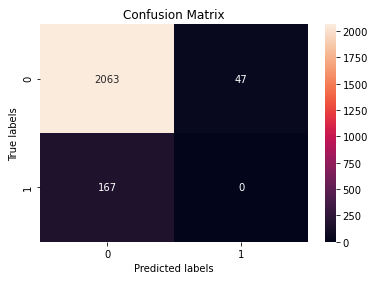# BCELossWithLogits Probability below 0.5

I am working on a binary classification problem for which I am using the BCELosswithLogits and (since my data is imbalanced) the BinaryFocalLoss. To get the probability of the sample being positive, I apply the sigmoid function to the output of the model. However, even though I could improve my model from 68% AUC to 81% AUC, when I look at the predicted probabilities, they are always below 0.5. Am I doing something wrong?

If the probabilities are always below 0.5, which I assume is the used threshold to get the predictions, I would also guess that the model is only predicting class0, i.e. the negative class in a binary classification setup?
If so, did you check the confusion matrix or other metrics?

Thanks for your reply! I will plot the confusion matrix but how do I know which is the correct threshold? So far, I assumed it is 0.5 but it could be different, couldn’t it?

`0.5` could be a good starting point and you could check the ROC for different thresholds and pick the best suitable one for your use case.

Thanks, I will try do to that as well.
So when using 0.5 as the threshold, I get the following confusion matrix:That means, that my model actually predicts both classes but it only predicts FP. So it doesn’t get any of the TP, correct? I don’t understand how that is possible. Do you think the threshold could affect this?

Yes, it seems that no FP are returned. Also yes, you can check the ROC to select the threshold for the TPR vs. FPR value you want.

I adjusted the threshold accordingly (it is 0.25). Does it make sense that my lowest probability is only 0.126 (I’d have assumed something closer to 0) and my highest (best predictions for TP) is still below 0.5? Should I not expect something close to 1?

The smaller range of values might indicate that the model wasn’t trained for many epochs and thus the logits have a relative narrow range. You could thus try to train the model for more epochs, in case it’s not overfitting.

Thanks for your reply! So far it is overfitting after more than 30 epochs but I will try to make further adjustments.